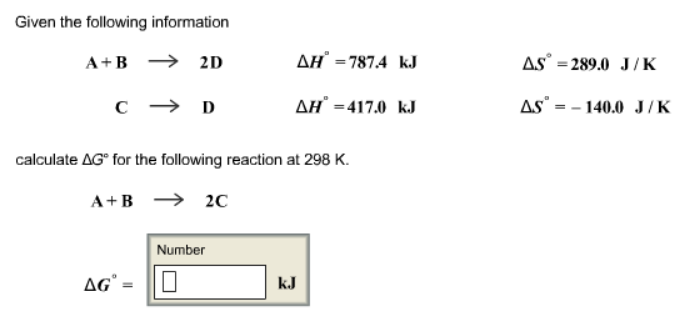# Problem: Given the following information A + B → 2D             ΔH° = 787.4 kJ              ΔS° = 289.0 J/K C → D                     ΔH° = 417.0 kJ               ΔS° = -140.0 J/K Calculate ΔG° for the following reaction at 298 K. A + B → 2C

###### FREE Expert Solution
90% (478 ratings)###### Problem Details

Given the following information

A + B → 2D             ΔH° = 787.4 kJ              ΔS° = 289.0 J/K

C → D                     ΔH° = 417.0 kJ               ΔS° = -140.0 J/K

Calculate ΔG° for the following reaction at 298 K.

A + B → 2C